# 22/7 circle

Calculate approximately the area of a circle with radius 20 cm. When calculating π, use 22/7.

S =  1257.14 cm2

### Step-by-step explanation: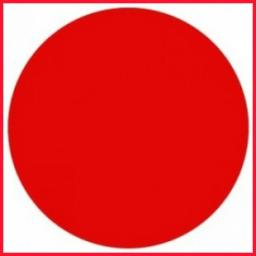We will be pleased if You send us any improvements to this math problem. Thank you!#### You need to know the following knowledge to solve this word math problem:

We encourage you to watch this tutorial video on this math problem:

## Related math problems and questions:

• Circle - easy 2The circle has a radius 6 cm. Calculate:Find the radius of the circle with area S = 200 cm².
• Bricks wallThere are 5000 bricks. How high wall thickness of 20 cm around the area which has dimensions 20 m and 15 m can use these bricks to build? Brick dimensions are 30 cm, 20 cm and 10 cm.
• Pavilion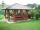The rectangular pavilion with dimensions 3.5 m and 2.75 m to be paved with square tiles of side 25 cm price of CZK 22 per 1 piece or rectangular tiles with sides of 20 cm and 15 cm in the price of CZK 11 per 1 pc. Which solution is cheaper (write its pric
• Diameter to areaFind the area of a circle whose diameter is 26cm.
• Circle area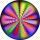Calculate the circle area with a radius of 1.2 m.Calculate the radius of the quadrant, which area is equal to area of circle with radius r = 15 cm.
• AnnulusThe radius of the larger circle is 8cm, the radius of smaller is 5cm. Calculate the contents of the annulus.
• Star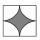Calculate the content of the shaded part of square with side 28 cm.
• Milk cartonsHow much paper do we need for 12 tetra packs with dimensions 6 cm, 11 cm, and 20 cm? Will 1 liter of milk fit in the box?
• Curved surface area CSA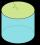A cylinder 5cm high has a base radius(7/2) cm. Calculate the curved surface area.
• Clocks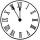What distance will describe the tip of minute hand 6 cm long for 20 minutes when we know the starting position with finally enclose hands each other 120°?
• Annulus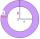Two concentric circles form an annulus of width 10 cm. The radius of the smaller circle is 20 cm. Calculate the content area of annulus.
• RegroupingSubtract mixed number with regrouping: 11 17/20- 6 19/20
• ParallelogramCalculate the area and perimeter of a parallelogram whose two sides are long a=24 cm b=22 cm and height ha = 6 cm long.
• Annulus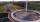Calculate the area of two circles annulus k1 (S, 3 cm) and k2 (S, 5 cm).
• Cloth / textileWe have cloth measure 16 square meters. How many 20 cm by 20 cm by 8 cm bags you can make? Assume bag is a cuboid without one top base.